## Definition

A tree T is a set of nodes that form a directed acyclic graph (DAG).

### Height

The height of a node ni is the length of the longest path under ni’s subtree.

• All leaves have a height of 0.

### Depth

The depth of a node ni is the length of the path from the root to ni.

• The root node has a depth of 0.
• The depth of a tree is the depth of its deepest leaf.

### Relationship

Height of Tree = Height of Root = Depth of Tree

## Implementation

1. Vecotr of Children
用 Vector 来保存所有子节点。这样做的好处是可以直接访问到每一个节点 node[i]，但是需要事先算好最多会有多少子节点来决定是否需要更多空间。

2. List of Children
用 List 来保存所有子节点。这样做可以有一个动态的长度；我们就不需要事先算好子节点的个数。但这样做也会增加访问每个节点嗦耗费的时间。

3. “Left-Child, Right-Sibling” or “First-Child, Next-Sibling”
以来类似链表的结构实现。这样会让每个节点都会维护两个指针分别指向其第一个子节点和下一个 兄妹 兄弟节点；我们就完全不需要担心子节点的个数了。但是同时访问时间也会变得与子节点的数量成正比。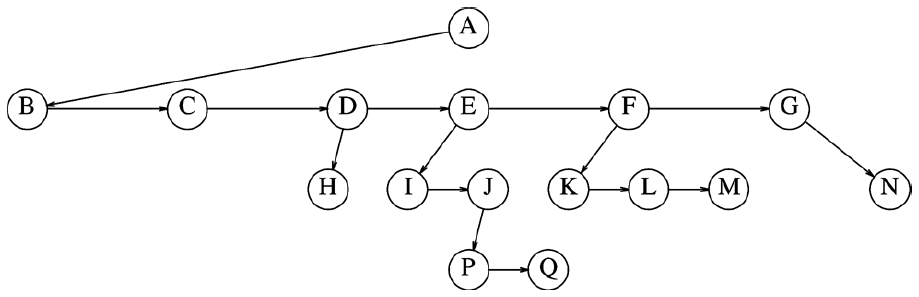## Binary Tree

A binary tree is a tree where each node has no more than two children.

## Binary Search Tree (BST)

### Deletion

1. 该节点没有任何子节点：直接移除它
2. 该节点有一个子节点：把子节点上移
3. 该节点有两个子节点：找到其最大子节点并上移

### Time Complexity

Complexity of searching a BST: $O(h)$ where h is the heigh of the tree.

Operations:

• Print Tree: $\Theta (N)$
• Intert, Remove, Search, Find Maximum Value and Find Minimum Value: $\Theta (h)$ where $h$ stands for height of the tree
• Worst case: $h = \Theta (N)$
• Best case: $h = \Theta (lgN)$
• Average case: $h = \Theta (lgN)$

## AVL (Adelson, Velskii and Landis)

AVL 树的主要理念为：

• 一个节点的左右子树的高度差最大为 1
• 在每次树被更新后强制维护整棵树
• 树的深度永远为 $O(log_2n)$

### Insert

Insertions can violate AVL’s balance, and this can be fixed by “rotation”.

• 新节点插入到了节点 k 的子节点的子树上
• 新节点插入到了节点 k 的子节点的子树上
• 新节点插入到了节点 k 的子节点的子树上
• 新节点插入到了节点 k 的子节点的子树上

#### Solution for Violation Case 1 (LL)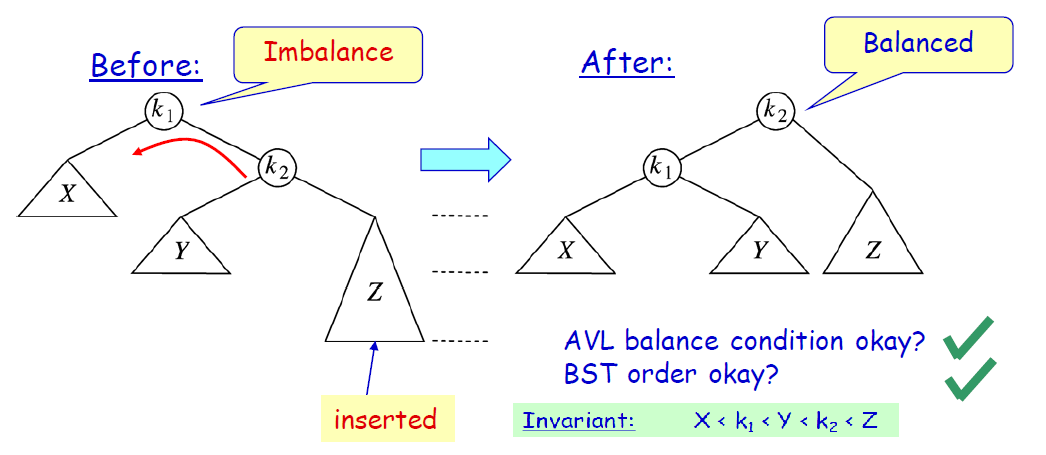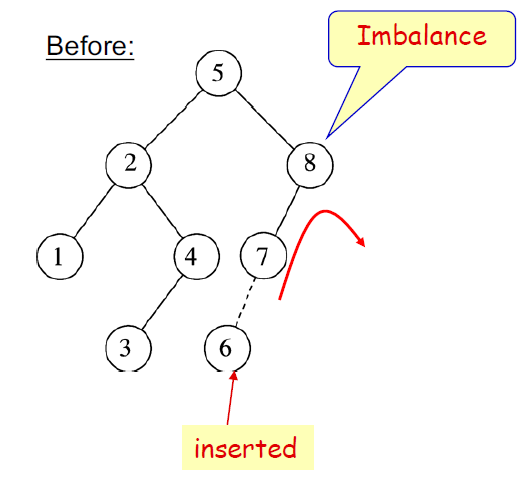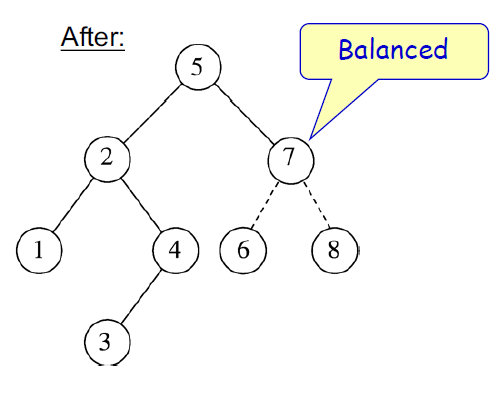#### Solution for Violation Case 4 (RR)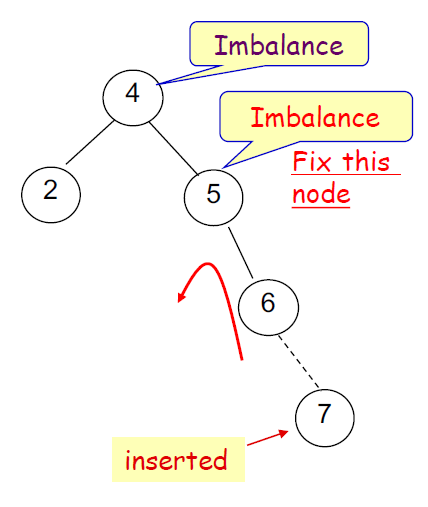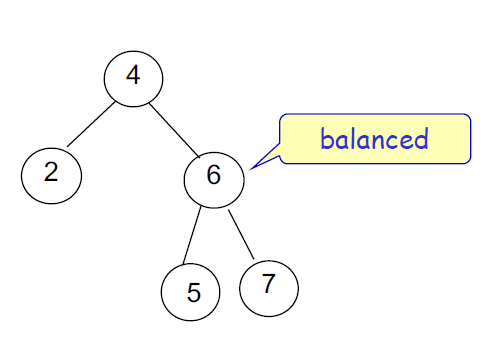#### Solution for Violation Case 2 (RL)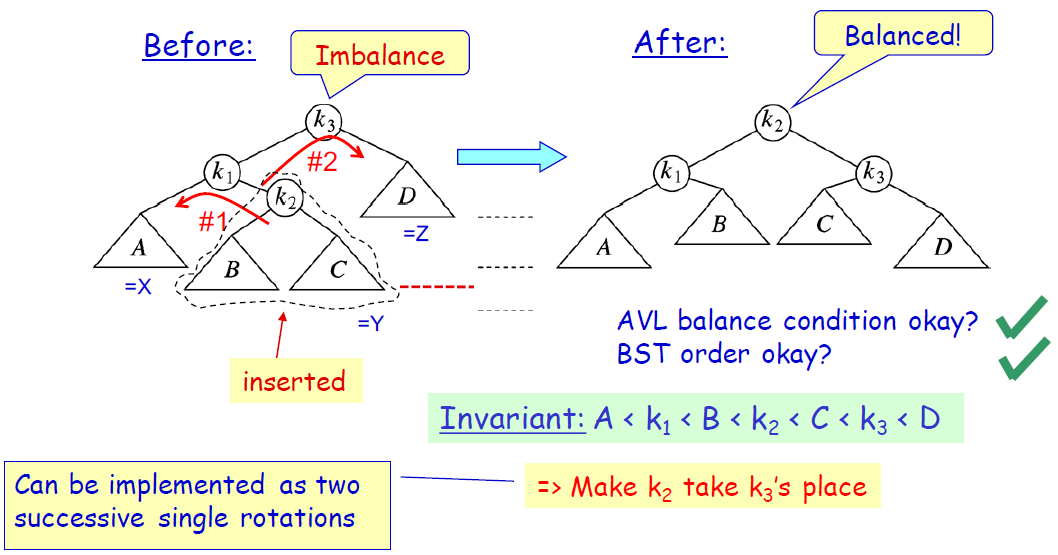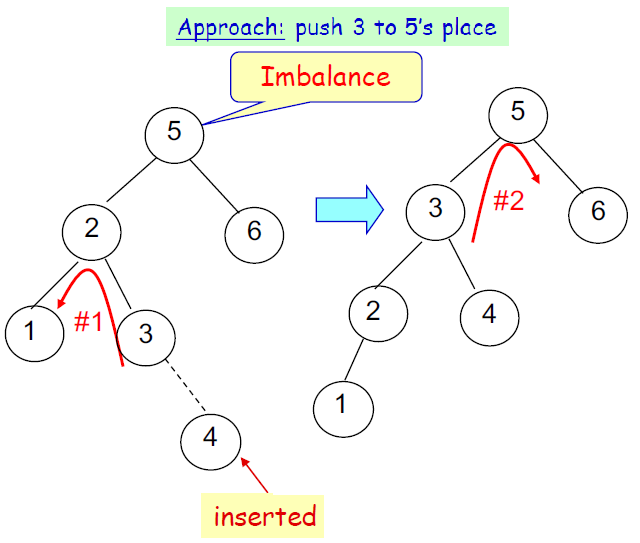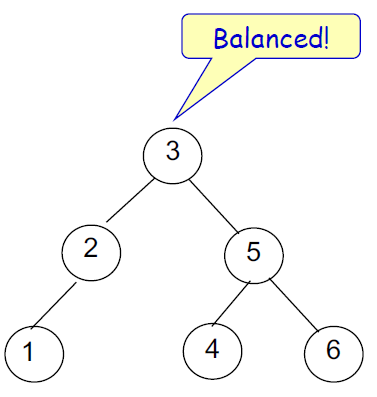#### Solution for Violation Case 3 (LR)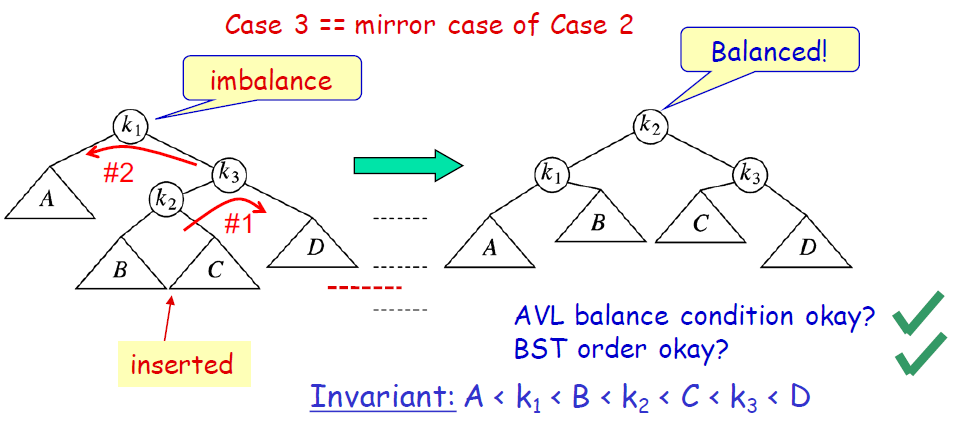In this case, the operation is the same as in case 2, but the order and direction are opposite.

To - Be - Done